# How to add / insert certain character every x characters into cells

When working with text data in Excel, it is possible that you will occasionally need to add the same text to cells that already exist in order to make things more understandable. For instance, you could want to insert a prefix at the beginning of each cell, a special symbol at the end, or specific text before a formula. You can do any of these things by using the Insert tab in Excel.

Everyone is familiar with how to complete this task manually. You will learn how to swiftly add strings containing a specified number of characters into the cell by following the below given VBA code in this tutorial.

Insert Certain Character between certain number of characters using VBA Code

Step 1

In our excel sheet we have some roll numbers in which we want to add the character “PHY” after 3 digits. See the below given image.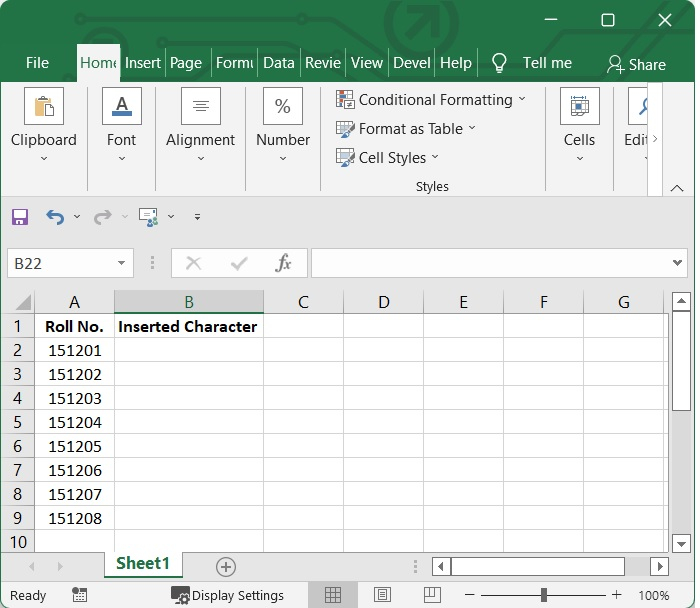Press Alt and F11 key (Alt+F11) to open Microsoft Visual Basic for Application windows. See the below given image.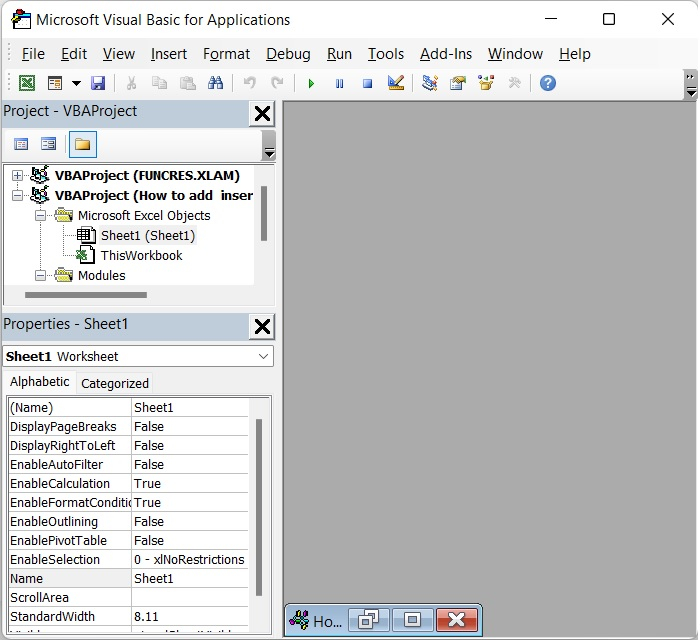Step 2

After that, select Insert > Module from the menu bar to bring up the popup Module window. See the below given image.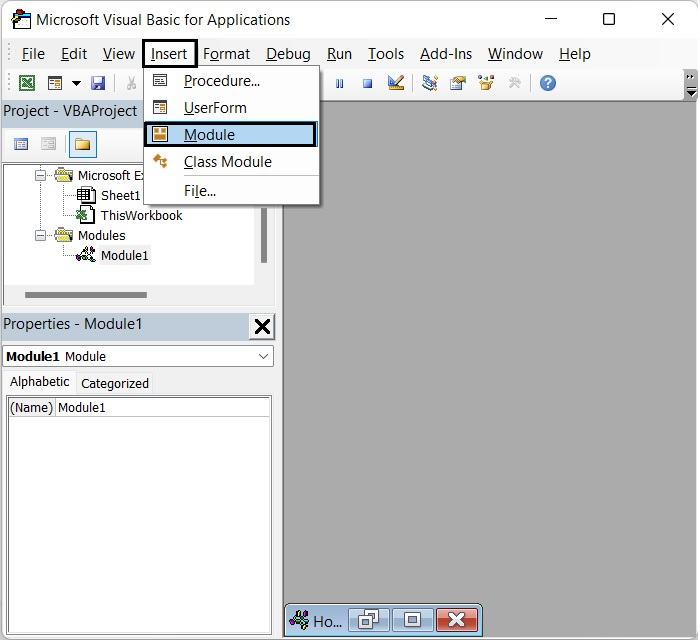Step 3

After opening the Module Window, then type the following VBA code in it.

Sub InsertCharacter()
Dim Rng As Range
Dim InputRng As Range, OutRng As Range
Dim xRow As Integer
Dim xChar As String
Dim index As Integer
Dim arr As Variant
Dim xValue As String
Dim outValue As String
Dim xNum As Integer
xTitleId = "VBOutput"
Set InputRng = Application.Selection
Set InputRng = Application.InputBox("Range :", xTitleId, InputRng.Address, Type:=8)
xRow = Application.InputBox("Number of characters :",
xTitleId, Type:=1)
xChar = Application.InputBox("Specify a character :", xTitleId, Type:=2)
Set OutRng = Application.InputBox("Out put to (single cell):", xTitleId, Type:=8)
Set OutRng = OutRng.Range("A1")
xNum = 1
For Each Rng In InputRng
xValue = Rng.Value
outValue = ""
For index = 1 To VBA.Len(xValue)
If index Mod xRow = 0 And index <> VBA.Len(xValue) Then
outValue = outValue + VBA.Mid(xValue, index, 1) + xChar
Else
outValue = outValue + VBA.Mid(xValue, index, 1)
End If
Next
OutRng.Cells(xNum, 1).Value = outValue
xNum = xNum + 1
Next
End Sub


See the below given image.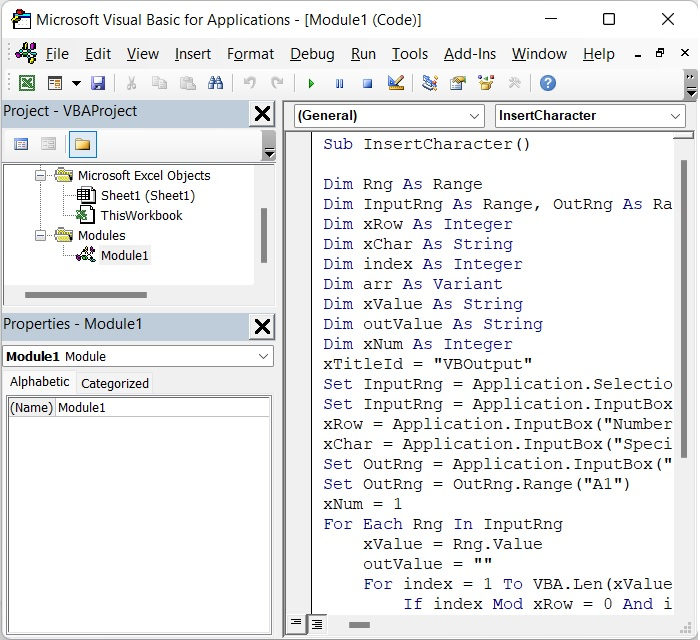Step 4

Then, press the F5 key to run this code. A box will pop up to remind you to choose the data range where you want to insert a certain character into the text strings, see the image given below. In our case we have chosen A2 :A9.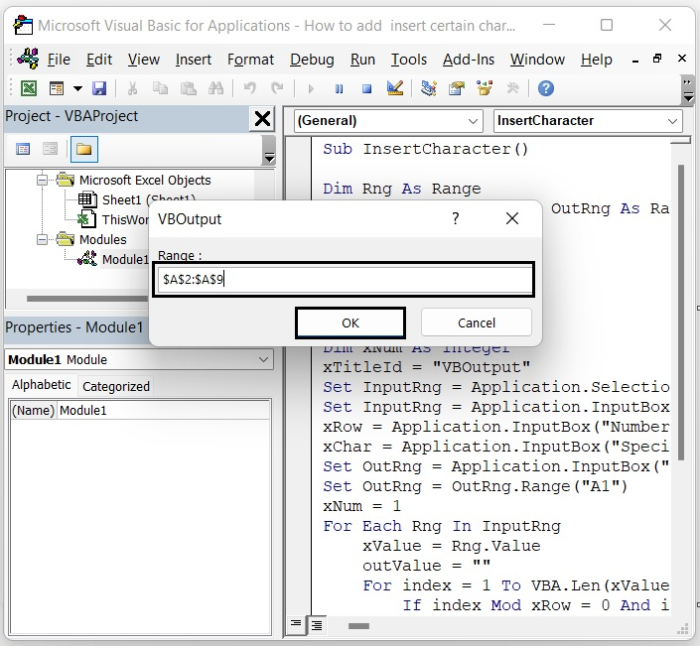Give the range as per your requirement and click OK.

Step 5

Another popup box will appear in which you have to insert the number which indicates after how many characters you want to insert the special character. In our case we have given 3.

See the following image.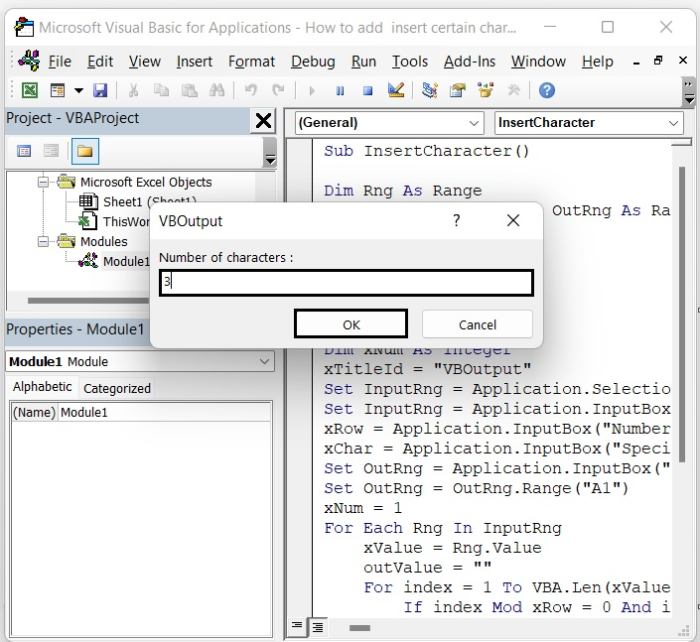Click OK after giving the number

Step 6

There will be another pop-up box where you would type the character you want to add to the text. In our case we have given the character as “PHY”.

See the following image.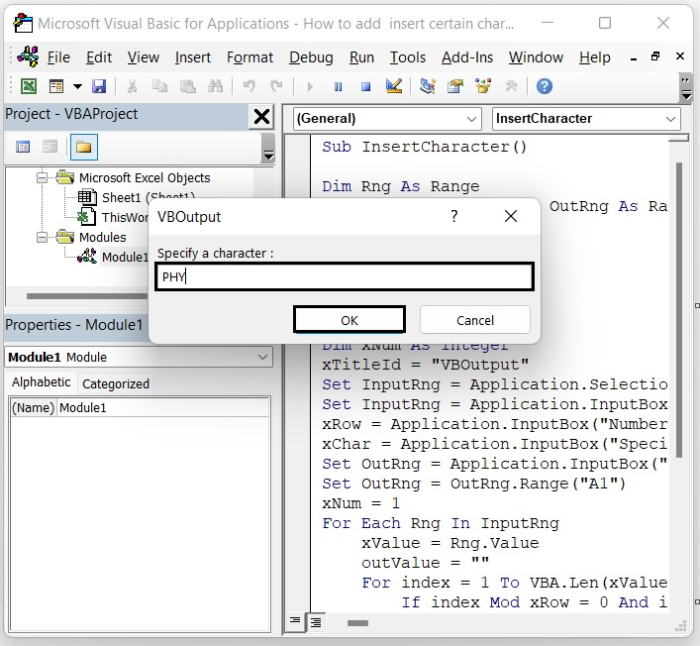Click OK after giving the Character.

Step 7

Another box will pop up, and you will have to type in the cell where you want the output result. In our case we have given B2 as the output result cell. See the following image.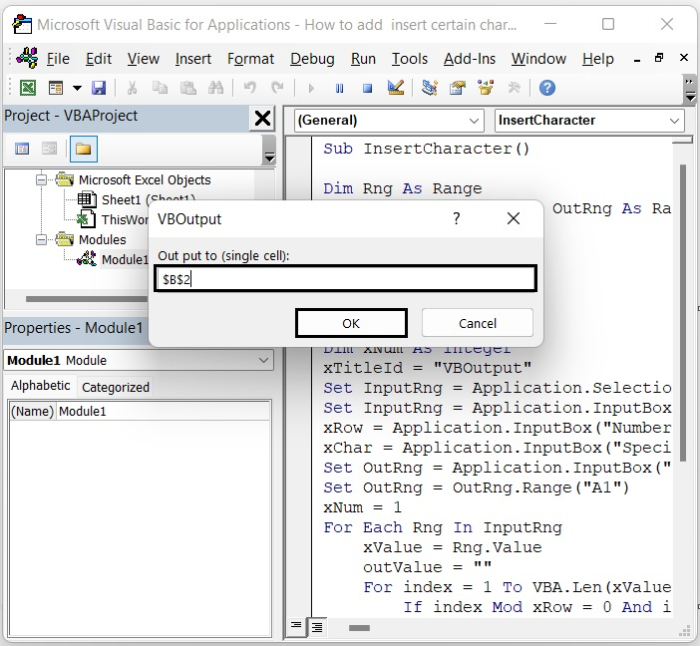Click OK after giving the output cell number.

You can see from the following image that the character “PHY” has been inserted into each text three characters. See the image given below.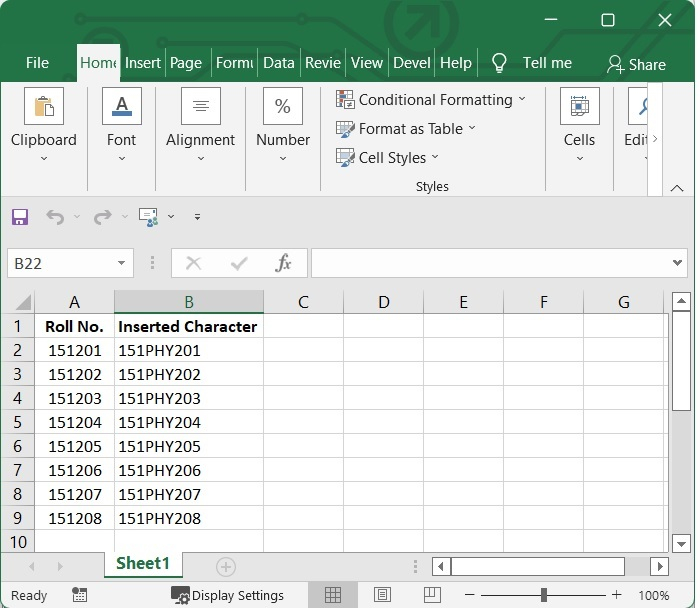Updated on: 12-Sep-2022

359 Views